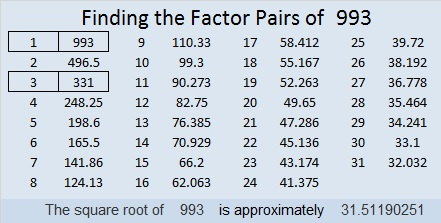993 Christmas Angel

There are 21 clues in this Christmas Angel puzzle. Will it be easy or difficult for you to solve? That is part of the mystery. As always, there is only one solution. Can you find it?Print the puzzles or type the solution in this excel file: 12 factors 993-1001

When is 993 a palindrome?
It is 5B5 in BASE 13 (B is 11 base 10) because 5(169) + 11(13) + 5(1) = 993,
313 in BASE 18 because 3(18²) + 1(18) + 3(1) = 993,
and it is repdigit 111 in BASE 31 because 31² + 31¹ + 31⁰ = 993

• 993 is a composite number.
• Prime factorization: 993 = 3 × 331
• The exponents in the prime factorization are 1 and 1. Adding one to each and multiplying we get (1 + 1)(1 + 1) = 2 × 2 = 4. Therefore 993 has exactly 4 factors.
• Factors of 993: 1, 3, 331, 993
• Factor pairs: 993 = 1 × 993 or 3 × 331
• 993 has no square factors that allow its square root to be simplified. √993 ≈ 31.5119This site uses Akismet to reduce spam. Learn how your comment data is processed.